Next: Jacobian matrix Up: Implementation Previous: Implementation   Contents

Return code

The procedure will return an integer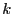•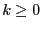: number of solutions
•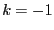: the size of the storage is too low ( possible solutions: increase M, or use the 3B method, or use the reverse storage mode or the single bisection mode)
•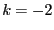: m or n is not strictly positive
•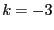: Order is not 0 or 1
•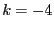: one of the function in the system has not a type 0, -1 or 1 (i.e. it's not an equation, an inequality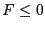or an inequality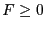)
•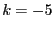: we are in the optimization mode and more than one functions are expressions to be optimized (see the Optimization chapter)
•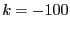: in the mixed bisection mode the number of variables that will be bisected is larger than the number of unknowns
•: one of the value of ALIAS_Delta3B or ALIAS_Max3B is negative or 0
•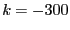: one of the value of ALIAS_SubEq3B is not 0 or 1
•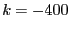: although ALIAS_SubEq3B has as size the number of equations none of its components is 1
•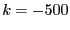: ALIAS_ND is different from 0 (i.e. we are dealing with a non-0 dimensional problem, see the corresponding chapter) and the name of the result file has not been specified
•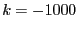: the value of the flag Single_Bisection is not correct
•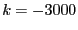: we use the full bisection mode and the problem has more than 10 unknowns
The following variables play also a role in the computation:
• ALIAS_Store_Gradient: if not 0 the gradient matrix of each box will be stored together with the input intervals. Must be set to 0 for large problem (default value: 1)
• ALIAS_Diam_Max_Gradient: if the maximal width of the ranges in a box is lower than this value, then the gradient will be used to perform the interval evaluation of the functions (default value: 1.e10)
• ALIAS_Diam_Max_Kraw: if the maximal width of the ranges in a box is lower than this value, then the Krawczyk operator will be used to determine if there is a unique solution in the box (default value: 1.e10)
• ALIAS_Diam_Max_Newton: if the maximal width of the ranges in a box is lower than this value, then the interval Newton method will be used either to try to reduce the width of the box or to to ensure that there is no solution of the system in the box (default value: 1.e10)Next: Jacobian matrix Up: Implementation Previous: Implementation   Contents
Jean-Pierre Merlet 2012-12-20